Structural Bioinformatics Library Template C++ / Python API for developping structural bioinformatics applications.
User Manual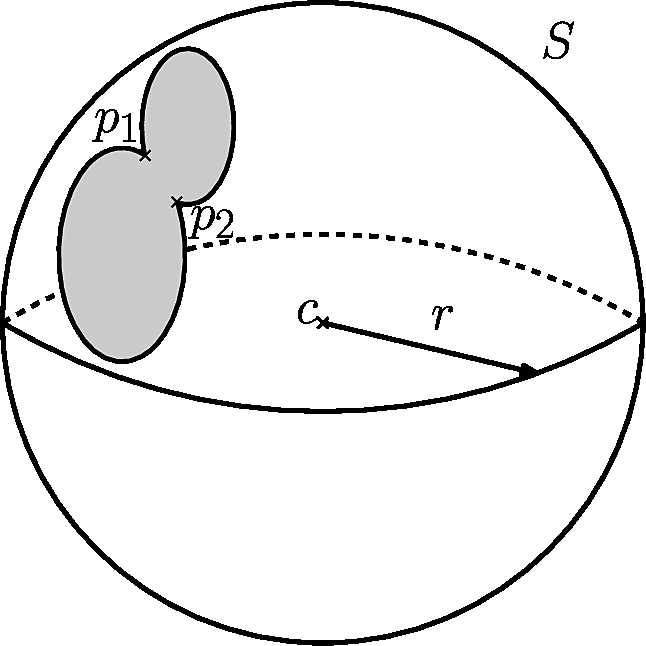# Spherical_kernel_extension_3

Authors: F. Cazals and T. Dreyfus

# Introduction

This package provides constructions and predicates related to the CGAL Spherical_kernel_3  . In particular, it allows to compute an orientation for the circular arcs. Given two spheres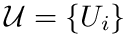,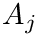that intersect on a circle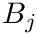, a point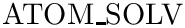on this circle and a circular arc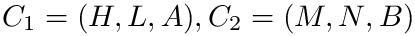starting atoriented with a tangent vector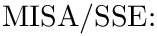at, we say thatis counter-clockwise oriented w.r.t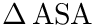iff the following mixed product is positive: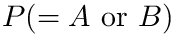. More intuitively, this means thatboundson its "left".

While the computation of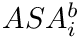is straightforward, computing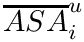andrequire managing degree two algebraic operations. To understand why, assume thatis counter-clockwise oriented:

• for, while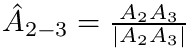has coordinates linearly computed from the coordinates and radii of the two spheres,belongs to the intersection circle and the coordinates may be degree two algebraic numbers, resulting on degree two algebraic numbers for the coordinates of;
• for, we can compute its from the cross product (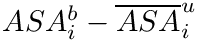), that results on a vector with degree two algebraic coordinates.

Now, given a third sphere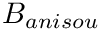, assume thatis the intersection point between,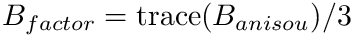and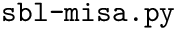, and consider the plane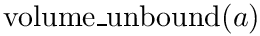tangent toat. Note thatcannot containnor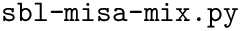. Sinceis the source of,andhave to point to different sides of. If it is not the case, this means thatis not counter-clockwise oriented.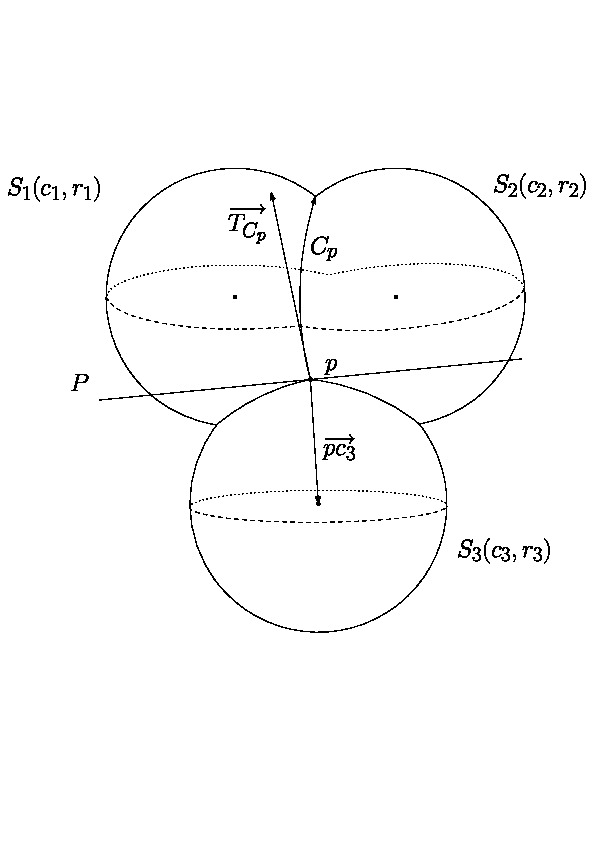We use these properties for determining the orientation of a circular arc defining by three intersecting spheres,and, and a reference point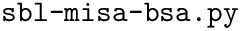allowing to locate the source of the circular arc:

•is the reference sphere for the orientation,
•anddefine the support circle of the circular arc,
•,,define at most two possible sources of the circular arc, anddetermines the side of the plane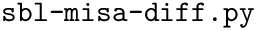that contains the source of the circular arc.

# Implementation

The SBL::GT::T_Spherical_kernel_extension_3 < SphericalKernel3 > is designed as a traits class defining the functors corresponding to the different constructions and predicates. The template parameter is one of the CGAL three dimensional spherical kernel, that could be the exact one (CGAL::Exact_spherical_kernel_3) or the generic one (CGAL::Spherical_kernel_3). See the Example section.

## Dependencies

This package has no internal dependency.

The only one external dependency is the CGAL library, for the representation of 3D geometric objects. Note that this part of the CGAL library is generic and consists only of C++ header files.

# Example

The following example presents the SBL::GT::T_Spherical_kernel_extension_3::Is_counter_clockwise_oriented predicate, with exact or inexact computations.

#include <iostream>
#include <fstream>
#include <SBL/GT/Spherical_kernel_extension_3.hpp>
#include <CGAL/Algebraic_kernel_for_spheres_2_3.h>
#include <CGAL/Exact_spherical_kernel_3.h>
//Kernel for representing geometric objects on a sphere, and for
//computations on a sphere.
typedef CGAL::Exact_spherical_kernel_3 SK;
//Extension of the Spherical Kernel.
int main(int argc, char *argv[])
{
//Creation of 3 spheres intersecting at two points.
SK::Sphere_3 S_1(SK::Point_3(0, 0, 0), 1);
SK::Sphere_3 S_2(SK::Point_3(1, 0, 0), 1);
SK::Sphere_3 S_3(SK::Point_3(1, -1, 0), 1);
//Creation of two points on each side of the plane containing the
//centers of the 3 spheres.
SK::Point_3 p_ref_1(0, 0, 1);
SK::Point_3 p_ref_2(0, 0, -1);
//Check that seing an oriented circular arc from a side of the plane
//containing the centers of the 3 spheres, is equivalent to see its
//opposite circular arc from the other side of the plane.
std::cout << "[(S_1, S_2, S_3), p_ref = (0, 0, 1)] vs [(S_2, S_1, S_3), p_ref = (0, 0, -1)]: "
<< Extension::Is_counter_clockwise_oriented()(S_1, S_2, S_3, p_ref_1)
<< " == "
<< Extension::Is_counter_clockwise_oriented()(S_2, S_1, S_3, p_ref_2)
<< std::endl;
return 0;
}